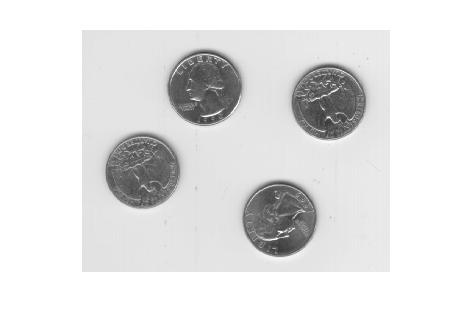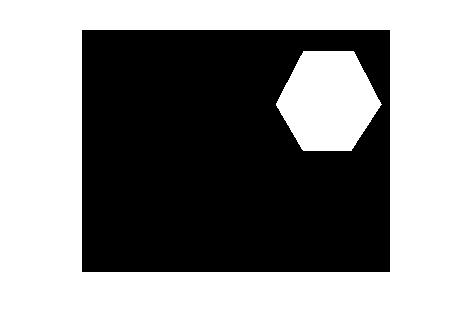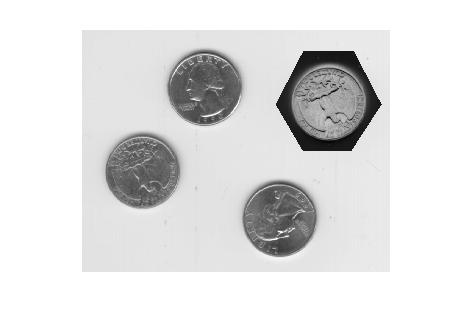ROIPOLY – rectangular regions and logical indexing

Several readers commented on my recent roipoly and poly2mask posts, and I wanted to follow up on a couple of issues they raised. The first issue is a common point of confusion about the selection of rectangular regions, and the second is how to access and modify pixels contained within a region of interest.

Rectangular regions

Users sometimes wonder why poly2mask and roipoly don't return some of the boundary pixels of a rectangular region as part of the mask. Here's an example.

x = [2 4 4 2 2];
y = [2 2 4 4 2];

0     0     0     0     0
0     0     0     0     0
0     0     1     1     0
0     0     1     1     0
0     0     0     0     0

Some users expect rows 2 through 4 and columns 2 through 4 to be included in the mask. One reader commented that he uses a different function instead of roipoly because of this behavior.

As I have illustrated in my previous posts, roipoly and poly2mask treat a pixel not as a point, but as a unit square area. The upper-left corner of the (2,2) pixel is (1.5,1.5), and the lower-right corner is (2.5,2.5). So the polygon defined by the x-y vertices in the example above covers only one-fourth of the (2,2) pixel.

I suggest that you get used to defining rectangles that cover complete pixel areas. Such rectangles go along pixel edges, not through pixel centers. Here's a second example to demonstrate:

xp = [1.5 4.5 4.5 1.5 1.5];
yp = [1.5 1.5 4.5 4.5 1.5];

0     0     0     0     0
0     1     1     1     0
0     1     1     1     0
0     1     1     1     0
0     0     0     0     0

Now the "mystery" is completely removed about which border rows and columns are in the mask. There's no need to use some other function.

Logical indexing

Another reader asked how to get and modify pixels inside a region of interest. One nice way to do that in MATLAB is to use logical indexing. The expression A(B), if B is logical and the same size as A, selects all the elements in A corresponding to the true elements of B.

I'll demonstrate using an example mask from the roipoly documentation.

clear
imshow(I)c = [222 272 300 270 221 194];
r = [21 21 75 121 121 75];If you use whos or look in the workspace browser, you can see that mask is a logical matrix.

whos
Name        Size             Bytes  Class      Attributes

I         242x308            74536  uint8
c           1x6                 48  double
r           1x6                 48  double

The expression I(mask) is a column vector containing all the elements of I corresponding to the true values of mask. Here, for example, is how you would compute the mean of the pixels inside the region of interest:

ans =

156.0468

You can use logical indexing on the left-hand side of an assignment as well.

I2 = I;
imshow(I2)Here's a documentation link for logical indexing. Or you might be interested in this MATLAB Digest article that Loren and I wrote about MATLAB indexing.

Published with MATLAB® 7.3

|